关于java引用传递的疑问？

年薪达不到25W大数据工程师、拿不到Offer全额退款->>>package yinyong;

public class Main {
public static void main(String[] args) {
A a = new A(null, "", "", "");
System.out.println(a);
function(a);
System.out.println(a);
}

static void function(A a) {
A a2 = new A("a", "b", "c", "d");
a = a2;//标注1
//a.setA(a2.getA());//标注2
}
}

A.java

package yinyong;

public class A {
String a;
String b;
String c;
String d;

public A() {
super();
}

public A(String a, String b, String c, String d) {
super();
this.a = a;
this.b = b;
this.c = c;
this.d = d;
}

public String getA() {
return a;
}

public void setA(String a) {
this.a = a;
}

public String getB() {
return b;
}

public void setB(String b) {
this.b = b;
}

public String getC() {
return c;
}

public void setC(String c) {
this.c = c;
}

public String getD() {
return d;
}

public void setD(String d) {
this.d = d;
}

@Override
public String toString() {
return "A [a=" + a + ", b=" + b + ", c=" + c + ", d=" + d + "]";
}

}

a = a2;//标注1

 A [a=null, b=, c=, d=] A [a=null, b=, c=, d=]

main方法中a的内容没有改变

a.setA(a2.getA());//标注2

 A [a=null, b=, c=, d=] A [a=a, b=, c=, d=]

main方法中a的内容有改变

 java中除了基本类型和String才是值传递，其他所有类型（包括 new String("xx")方式创建的字符串）都是引用传递。

1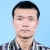// Java只有值传递
a = a2;//a2和a指向同一块值存储，没有改变原有a对象,作用域为方法
a.setA(a2.getA());//通过自定义属性更改方法改变了a对象，打印出来的hash变化了
// toString源码
public String toString{
return getClass().getName() + "@" + Integer.toHexString(hashCode());
}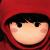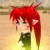java确实只有值传递，除了基本类型，你看似引用传递的对象，其实传递的是引用的地址值，我是搬运工http://www.cnblogs.com/coderising/p/5697986.htmla=a2 这个应该使a指向了a2的物理地址吧，和本来a的物理地址不同，我debug的时候可以看到a的内容已经变了，但运行完这个function在main中a是没有变化了，就像您说的作用域是方法内，请问为什么会这样呢？
1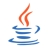java的引用不是变量名的引用，而是说变量存储的内容是指针。

1

1
J

J1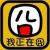http://blog.csdn.net/fbysss/article/details/3082949，一篇老文章，但对Java的参数传递解释得很清楚。function 中的a是传递进去的参数的一个副本a'，因此对这个副本a‘的操作不影响函数体外部的参数 。1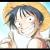“在Java里面参数传递都是按值传递”这句话的意思是：按值传递是传递的值的拷贝，按引用传递其实传递的是引用的地址值，所以统称按值传递。

A{field1='null', field2='', field3='', filed4=''}
A{field1='null', field2='', field3='', filed4=''}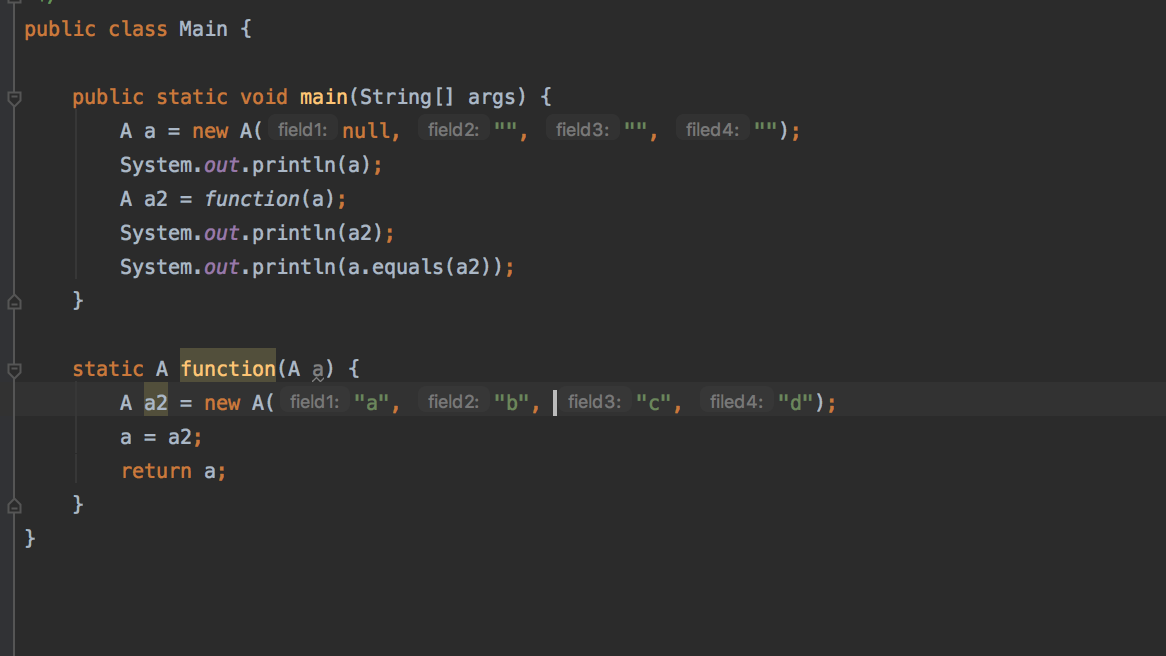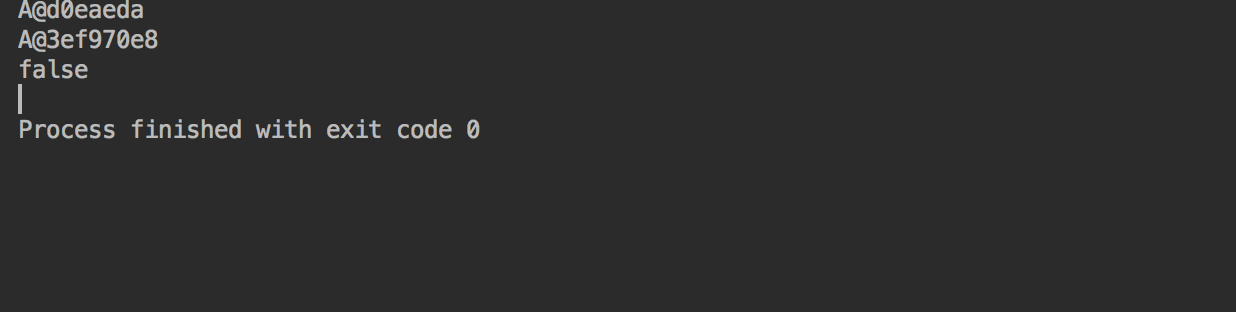1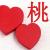0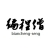a=a2:创建一个引用变量a2,将 a2的地址赋给了形参a（方法中的形参a，方法结束释放资源，不影响main中的变量）,所以a输出前后没有改变；

a.setA(a2.getA())：执行该操作是将a2地址指向的值取出来存到a地址指向的内存，a对象地址没有改变，但是地址指向内存中的值变了.

0
0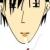java都是值传递  引用传递是传递地址